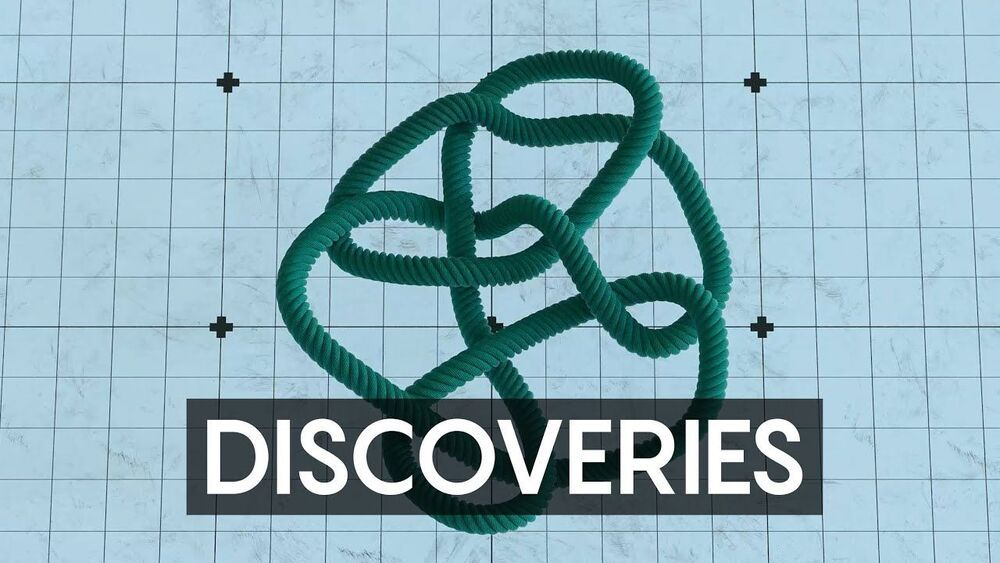## БЛОГFor mathematicians and computer scientists, 2020 was full of discipline-spanning discoveries and celebrations of creativity. We’d like to take a moment to recognize some of these achievements.

1. A landmark proof simply titled MIP = RE” establishes that quantum computers calculating with entangled qubits can theoretically verify the answers to an enormous set of problems. Along the way, the five computer scientists who authored the proof also answered two other major questions: Tsirelson’s problem in physics, about models of particle entanglement, and a problem in pure mathematics called the Connes embedding conjecture.

2. In February, graduate student Lisa Piccirillo dusted off some long-known but little-utilized mathematical tools to answer a decades-old question about knots. A particular knot named after the legendary mathematician John Conway had long evaded mathematical classification in terms of a higher-dimensional property known as sliceness. But by developing a version of the knot that yielded to traditional knot analysis, Piccirillo finally determined that the Conway knot is not slice.

3. For decades, mathematicians have used computer programs known as proof assistants to help them write proofs — but the humans have always guided the process, choosing the proof’s overall strategy and approach. That may soon change. Many mathematicians are excited about a proof assistant called Lean, an efficient and addictive proof assistant that could one day help tackle major problems. First, though, mathematicians must digitize thousands of years of mathematical knowledge, much of it unwritten, into a form Lean can process. Researchers have already encoded some of the most complicated mathematical ideas, proving in theory that the software can handle the hard stuff. Now it’s just a question of filling in the rest.